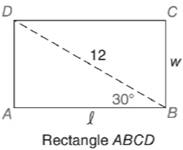Chapter 11.3, Problem 20E### Elementary Geometry for College St...

6th Edition
Daniel C. Alexander + 1 other
ISBN: 9781285195698

#### Solutions

Chapter
Section### Elementary Geometry for College St...

6th Edition
Daniel C. Alexander + 1 other
ISBN: 9781285195698
Textbook Problem
1 views

# In Exercises 15 to 20, use the sine, cosine, or tangent ratio to find the lengths of the indicated sides to the nearest tenth of a unit.To determine

To find:

The lengths of the indicated sides to the nearest tenth of a unit using the sine, cosine or tangent ratio.

Explanation

Given:

The rectangle ABCD is shown below.

Definition used:

The trigonometric ratios:

Calculation:

Since ABCD is a rectangle, BC=AD.

Let α=30.

We need to find the value of w, l.

We have sinα=oppositehypotenuse

sin30=w12

cos30=l12

Let us use Ti-83 calculator to find the value of sin30,cos30.

Press ON in Ti-83 calculator.

Since we need to find the value of degree, we need to change the mode to degrees.

Press MODE button and use arrow keys to select the “Degree” mode.

Let us find the values, correct to four decimal places.

Since we need the values of sin30,cos30 correct to four decimal places, in MODE, using arrow keys choose the value of “Float” as 4

### Still sussing out bartleby?

Check out a sample textbook solution.

See a sample solution

#### The Solution to Your Study Problems

Bartleby provides explanations to thousands of textbook problems written by our experts, many with advanced degrees!

Get Started

#### Convert the expressions in Exercises 6584 to power form. 35x5x8+72x3

Finite Mathematics and Applied Calculus (MindTap Course List)

#### Factor each expression in Problems 7-18 as a product of binomials. 13.

Mathematical Applications for the Management, Life, and Social Sciences

#### Graphing Inequalities 10. y 3x

Precalculus: Mathematics for Calculus (Standalone Book)

#### True or False: If h(x) = g(x) for all x a and limxah(x)=L, then limxag(x)=L.

Study Guide for Stewart's Single Variable Calculus: Early Transcendentals, 8th